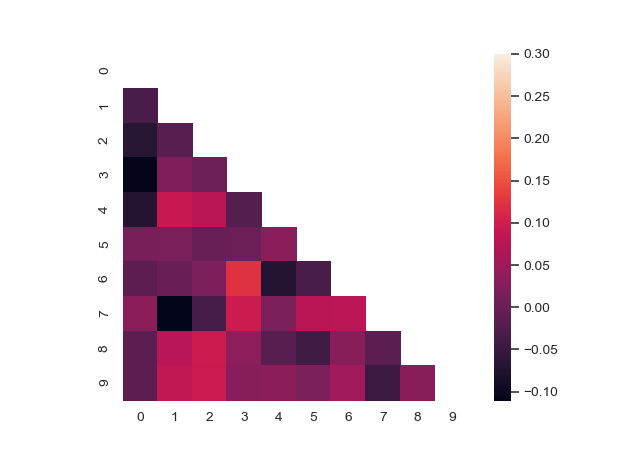# seaborn.heatmap¶

`seaborn.``heatmap`(data, vmin=None, vmax=None, cmap=None, center=None, robust=False, annot=None, fmt='.2g', annot_kws=None, linewidths=0, linecolor='white', cbar=True, cbar_kws=None, cbar_ax=None, square=False, xticklabels='auto', yticklabels='auto', mask=None, ax=None, **kwargs)

Plot rectangular data as a color-encoded matrix.

This is an Axes-level function and will draw the heatmap into the currently-active Axes if none is provided to the `ax` argument. Part of this Axes space will be taken and used to plot a colormap, unless `cbar` is False or a separate Axes is provided to `cbar_ax`.

Parameters
datarectangular dataset

2D dataset that can be coerced into an ndarray. If a Pandas DataFrame is provided, the index/column information will be used to label the columns and rows.

vmin, vmaxfloats, optional

Values to anchor the colormap, otherwise they are inferred from the data and other keyword arguments.

cmapmatplotlib colormap name or object, or list of colors, optional

The mapping from data values to color space. If not provided, the default will depend on whether `center` is set.

centerfloat, optional

The value at which to center the colormap when plotting divergant data. Using this parameter will change the default `cmap` if none is specified.

robustbool, optional

If True and `vmin` or `vmax` are absent, the colormap range is computed with robust quantiles instead of the extreme values.

annotbool or rectangular dataset, optional

If True, write the data value in each cell. If an array-like with the same shape as `data`, then use this to annotate the heatmap instead of the data. Note that DataFrames will match on position, not index.

fmtstring, optional

String formatting code to use when adding annotations.

annot_kwsdict of key, value mappings, optional

Keyword arguments for `ax.text` when `annot` is True.

linewidthsfloat, optional

Width of the lines that will divide each cell.

linecolorcolor, optional

Color of the lines that will divide each cell.

cbarboolean, optional

Whether to draw a colorbar.

cbar_kwsdict of key, value mappings, optional

Keyword arguments for fig.colorbar.

cbar_axmatplotlib Axes, optional

Axes in which to draw the colorbar, otherwise take space from the main Axes.

squareboolean, optional

If True, set the Axes aspect to “equal” so each cell will be square-shaped.

xticklabels, yticklabels“auto”, bool, list-like, or int, optional

If True, plot the column names of the dataframe. If False, don’t plot the column names. If list-like, plot these alternate labels as the xticklabels. If an integer, use the column names but plot only every n label. If “auto”, try to densely plot non-overlapping labels.

If passed, data will not be shown in cells where `mask` is True. Cells with missing values are automatically masked.

axmatplotlib Axes, optional

Axes in which to draw the plot, otherwise use the currently-active Axes.

kwargsother keyword arguments

All other keyword arguments are passed to `matplotlib.axes.Axes.pcolormesh()`.

Returns
axmatplotlib Axes

Axes object with the heatmap.

`clustermap`

Plot a matrix using hierachical clustering to arrange the rows and columns.

Examples

Plot a heatmap for a numpy array:

```>>> import numpy as np; np.random.seed(0)
>>> import seaborn as sns; sns.set()
>>> uniform_data = np.random.rand(10, 12)
>>> ax = sns.heatmap(uniform_data)
```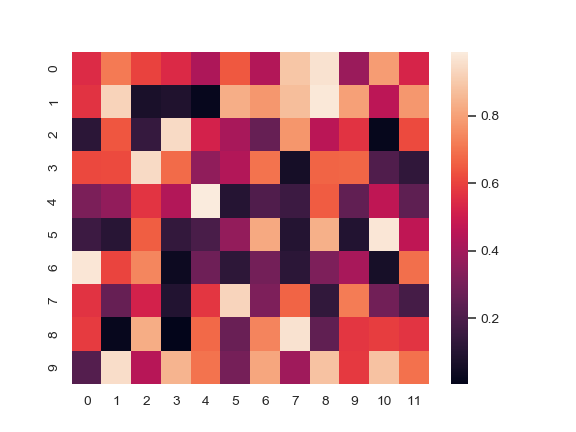Change the limits of the colormap:

```>>> ax = sns.heatmap(uniform_data, vmin=0, vmax=1)
```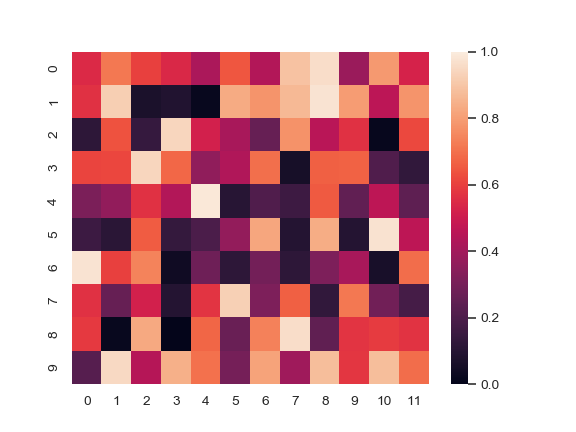Plot a heatmap for data centered on 0 with a diverging colormap:

```>>> normal_data = np.random.randn(10, 12)
>>> ax = sns.heatmap(normal_data, center=0)
```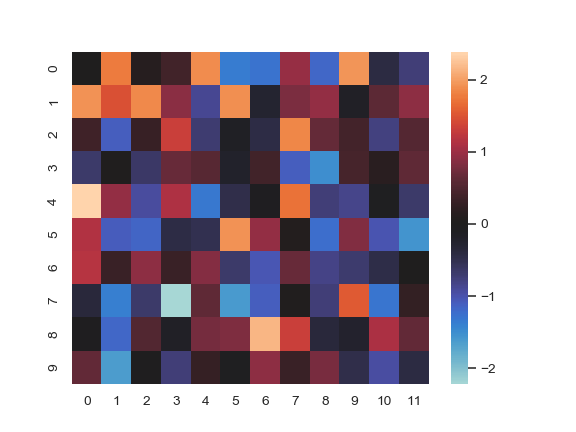Plot a dataframe with meaningful row and column labels:

```>>> flights = sns.load_dataset("flights")
>>> flights = flights.pivot("month", "year", "passengers")
>>> ax = sns.heatmap(flights)
```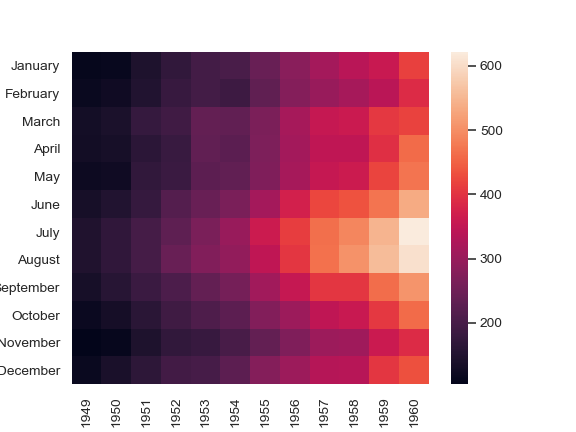Annotate each cell with the numeric value using integer formatting:

```>>> ax = sns.heatmap(flights, annot=True, fmt="d")
```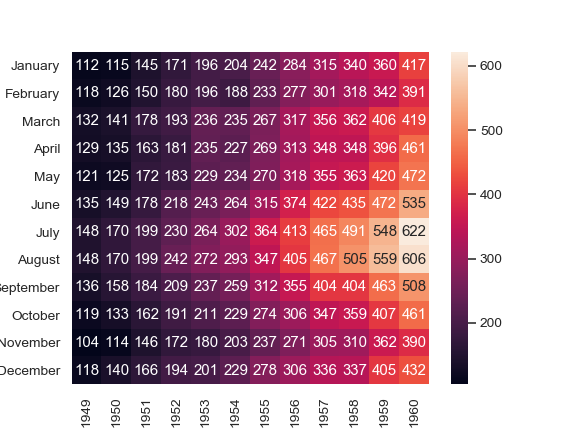```>>> ax = sns.heatmap(flights, linewidths=.5)
```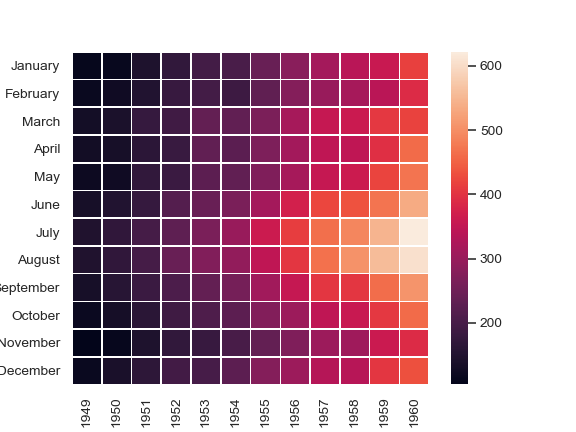Use a different colormap:

```>>> ax = sns.heatmap(flights, cmap="YlGnBu")
```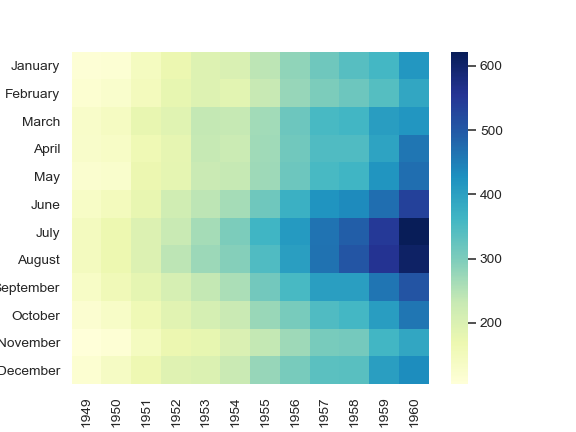Center the colormap at a specific value:

```>>> ax = sns.heatmap(flights, center=flights.loc["January", 1955])
```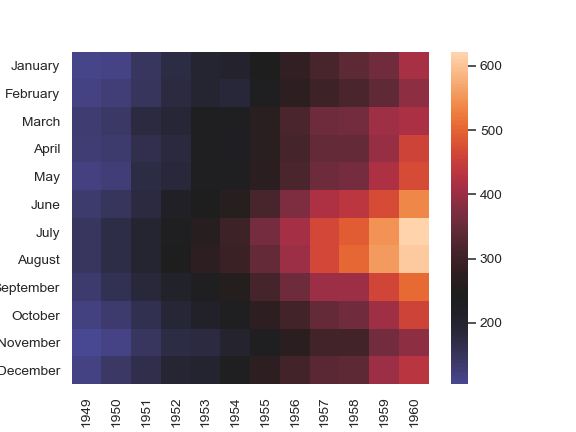Plot every other column label and don’t plot row labels:

```>>> data = np.random.randn(50, 20)
>>> ax = sns.heatmap(data, xticklabels=2, yticklabels=False)
```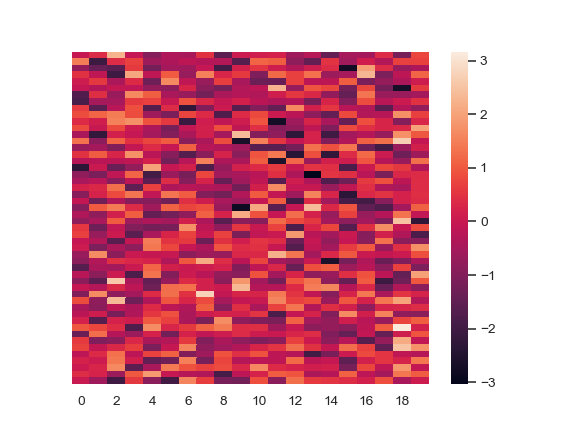Don’t draw a colorbar:

```>>> ax = sns.heatmap(flights, cbar=False)
```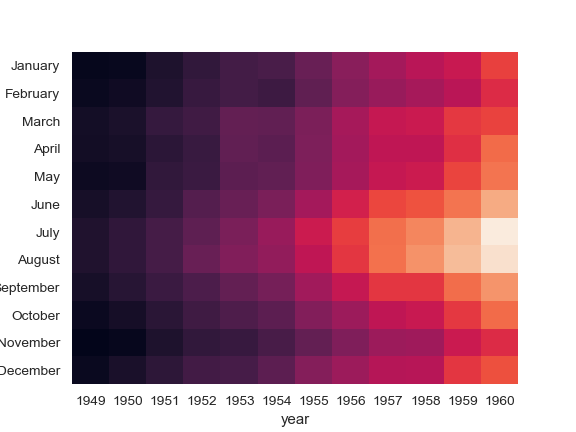Use different axes for the colorbar:

```>>> grid_kws = {"height_ratios": (.9, .05), "hspace": .3}
>>> f, (ax, cbar_ax) = plt.subplots(2, gridspec_kw=grid_kws)
>>> ax = sns.heatmap(flights, ax=ax,
...                  cbar_ax=cbar_ax,
...                  cbar_kws={"orientation": "horizontal"})
```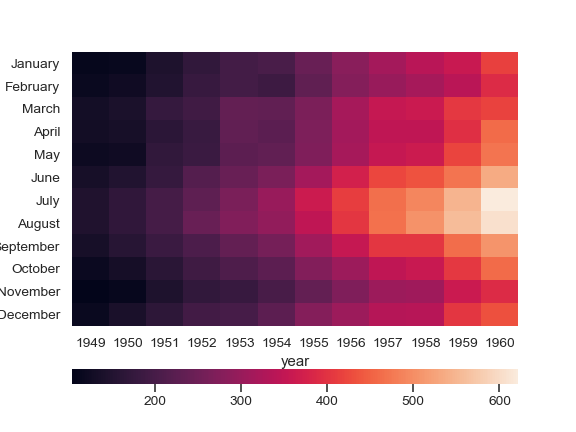Use a mask to plot only part of a matrix

```>>> corr = np.corrcoef(np.random.randn(10, 200))